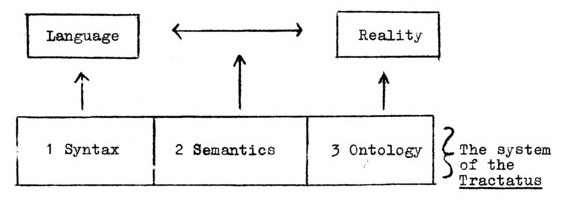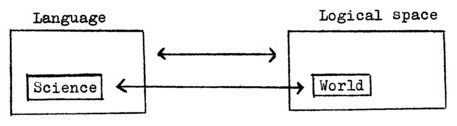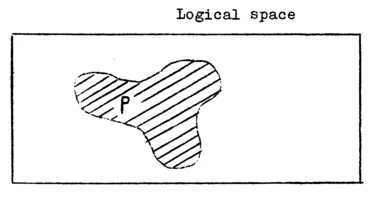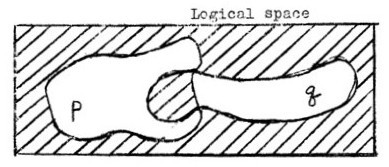Wittgensteinian Foundations of Non‑Fregean Logic

Boguslaw Wolniewicz

The term "Non‑Fregean Logic" has been introduced in 1968 by the well‑known Polish logician Professor Roman Suszko to mark the distinction between two kinds of logical systems. A logical system is called by him "Fregean", if for its propositional calculus the following formula holds as a theorem:

(F)       P ≡ q →  P = q

He calls this formula "the axiom of Frege, and it is not difficult to see why. (According to Frege's theory of meaning all true propositions denote the same, and similarly—all false ones. Thus if two propositions are materially equivalent, their denotations have to be identical; and exactly this is stated by the formula F.) The foremost example of a Fregean logic is the classical prepositional calculus, but—as we shall see—the three‑valued logic of Łukasiewicz is Fregean too. On the other hand, a logical system is called "Non‑Fregean" if the formula F is rejected in it as a theorem.

Some logicians have objected to drawing any such distinction, on the ground that in the classical propositional calculus there is no identity sign for propositions, and that consequently nothing like the "axiom of Frege" can be a theorem of this particular logical system. This objection, however, has a flavor of spurious innocence, being apparently based on the dubious principle that what is not spoken about doesn't exist. To see this let's note in the first place that the axiom of Frege is deductively equivalent to the following schematic formula:

(F' )      P ≡ q → / Φ (p) ≡ Φ (q) /

Certainly, in the ordinary propositional calculus there is no such schema either. (Though it is present in one of its variants, namely in Leśniewski's protothetics.) But we have there all its particular instances, known as "the laws of extensionality":

p ≡ q → ~p ≡ ~q

p ≡ q → p ∧ r=q ∧ r

and so on.

By these laws whatever holds good of one of two materially equivalent propositions, holds also of the other one. Thus from the point of view of classical propositional logic equivalent propositions are indistinguishable, and being indistinguishable they are by Leibniz's principle identical. This is, however, only another way of stating the axiom of Frege, so what is the point of objecting to it?

The idea of a Non‑Fregean logic goes back to Wittgenstein's Tractatus, where it is introduced right from the start in the thesis:

"1.13 Facts in logical space are the world."

The Tractatus is in the first place a work on the philosophy of logic, and the key to that philosophy is the concept of "logical space". Part of that concept is the idea of a Non‑Fregean logic.

Before going on, something has to be said here concerning Wittgenstein's general philosophical position. In our Marxist literature it is a firmly established opinion that Wittgenstein was a logical positivist, and that consequently his philosophical outlook is that of subjective idealism. This opinion, however, doesn't bear scrutiny. In fact it has been rashly taken over from the logical positivists of the Vienna Circle, whose early enthusiasm for the Tractatus was only a sad monument of misunderstanding and a rare specimen of philosophical blindness. Wittgenstein's doctrine is idealistic, no doubt about that. But its idealism is not of the subjectivist variety characteristic of positivism. The doctrine of the Tractatus is a peculiar and powerful variant of objective idealism, and it has much more in common with the doctrines of Plato or Leibniz, than with those of Berkeley and Mach. This again is most readily seen while investigating the philosophical import of the concept of "logical space".

The Tractatus starts from the assumption that the logic of language—its logical syntax—has been already, and in the main correctly, described by the systems of Frege and Russell. But there still remains the big question of a correct interpretation of that description, and it may be put as follows: the logical structure of language being such as described by Frege and Russell, what must be the ontological structure of a reality capable of being described by such a language?

Wittgenstein's answer to this question is embedded in the whole system of the Tractatus, and it may be useful to represent its framework schematically in a simple diagram (due to Suszko):It is fairly easy to discern in the text of the book particular theses forming the three main parts of its system; e.g. thesis 4.22. "An elementary proposition consists of names" surely belongs to part (1), 3.203: "The name denotes an object", — to part (2), and 2.02: "The object is simple" — to part (3). Thesis 1.13 is obviously an ontological one too, as are, by the way, all the theses numbered 1 ‑ 2.0. . ..

Now according to Wittgenstein's syntax, language is the totality of propositions, and according to his semantics the correspondence between language and reality has to be of the one‑to‑one type. What then, according to his ontology, is meant here by reality, the one‑to‑one counterpart of the totality of propositions? Reality cannot be identical with the world, for the world is the totality of facts, and to the totality of facts there corresponds in language only the totality of true propositions (= science, 4.11). Since language contains also false propositions, and these do not have counterparts in the totality of facts, it proves to be larger than the world; and the same holds good of reality too. If the overall semantical correspondence is to be preserved, something in reality must answer even to a false proposition. (And it has to be preserved, for if nothing in reality answered to false propositions they would have no relation to it; and being thus out of touch with reality they could not be false, but only meaningless.)

According to the Tractatus the ontological counterpart of a true proposition is a fact; and the ontological counterpart of a false proposition is the possibility of a fact, something that might be the case. (On the other hand, any fact is the actualization of some possibility.) And reality is the totality of all possibilities called by Wittgenstein "logical space". Thus we have the following identities:

Language = the totality of propositions,

Science = the totality of true propositions,

The world = the totality of facts,

Logical space = the totality of possibilities;

and to make their relations even more precise we may present them in the form of a diagram:The meaning of Wittgenstein's pronouncement in 1.13 is sufficiently clear now: the world is, so to speak, an island of facts in the ocean of possibilities. But this simile is only a useful first approximation to Wittgenstein's idea of logical space. And we proceed now to the second one.

Logical space is the ontological counterpart of language taken as a whole, but what are the counterparts to its particular propositions? According to Wittgenstein to every proposition there corresponds a definite area of logical space, or—as he calls ita logical place. Thus the logical place of a given proposition "p" may be visualized like this:It is already apparent that Wittgenstein's idea aims at the construction of a geometrical representation for the logic of propositions, and that his "logical space" is an abstract space like the "phase‑space" of physics or the "sample‑space" of the theory of probability. And this leads immediately to the next and most essential question: what are to be the points of this abstract logical space?

The right answer: to this question has been already given by Stenius (Wittgenstein's 'Tractatus', 1960): every point in logical space is the representation of a possible world! (Stenius' answer is not the only one that has been suggested, but none of the others will do as an interpretation of Wittgenstein's position.) Let's call these worlds "logical points". We have thus:

Logical space = the totality of logical points,

The logical             the set of logical points which
place of p"    =     would make the proposition "p" true.

One point in logical space is designated: it represents the actual world. (Since each possible world is incompatible with every other the designated point is unique.) Of course, we do not know its exact position; but if we know a proposition "p" to be true, we know the designated point to lie in that area of logical space which is the logical place of "p". Thus we have:

"p" is true = the designated point is contained in the logical place of "p".

According to Frege the denotation of a proposition is its truth‑value; according to Wittgenstein the denotation of a proposition is its logical place (= a set of possible worlds). And 'this makes clear, why formula (F) has to be rejected.

A material equivalence "p ≡ q" means that the propositions p and q have the same truth-value. Upon our interpretation this corresponds to the following situation in logical space:A statement of material equivalence "p ≡ q" is true if, and only if. the designated point lies as a matter fact [sic] somewhere in the shaded area of logical space. And this may be the case, or it may not be. On the other hand, an identity statement "p = q means that the logical places of "p" and "q" coincide; and this cannot be the case here by any means.

But how can formula (F) be a theorem of "Fregean" logic, if it is not valid? To solve this puzzle let us assume for the sake of intuitiveness that there are only three possible worlds, marked by the numbers (1), (2), (3) respectively. Under this assumption the relation between language and reality may be presented in the form of a matrix, with columns of the digits "1" and "0" representing the logical places of the corresponding propositions:

Language

p1   p2   p3   p4   p5   p6   p7   p8

(1)       1    1     1      1     0     0     0     0

Logical space   (2)       1    1     0      0     1     1     0     0

(3)       1    0     1      0     1     0     1     0

In the logical space consisting of only 3 points there are only 8 logical places; and consequently there are in the corresponding language only 8 extensionally distinguishable propositions (i.e. propositions with different denotations).

However, our assumption was quite arbitrary, for there are as yet no obvious reasons against taking any other number—finite or infinite—to be the number of logical points. So let us assume now, that this number is one: There is only one possible world, namely the actual one. Under this assumption we get the following matrix of the relation between language and reality:

Language

p1    p2

Logical space {  (1)   1     0

In this matrix there are only two logical places. And since these two logical places (columns) are correlated in a one‑to‑one manner with the two truth‑values (digits), there is neither the need nor the possibility of distinguishing the logical place of a proposition from its truth‑value, or the designated truth‑value from the designated logical point.

But this is exactly the import of the axiom of Frege. According to Frege true propositions are indistinguishable extensionally, for they all denote (bedeuten) one and the same, namely the real world or Being (das Wahre); and similarly with false propositions: they all denote Non‑Being (das Falsche). Therefore Being and Non‑Being are the two logical places of Frege's logic.

The number of logical places (m) depends obviously upon the number of truth‑values (v), and upon the number of logical points (n); and they are interrelated in a most simple way:

(I)        m=vⁿ

If our logic is, as usual, a two‑valued one (v = 2), clearly the number of logical places will be: m = 2ⁿ. But to Fregean logic it is not essential to assume that there are only two truth‑values. What is essential to it, is to have the equality:

(II)       m = v

which in view of (I) is equivalent to assuming that the number of logical points is one! i.e.:

(F'')     n = 1

Formula (F) holds good if, and only if, condition (F'') is satisfied. In other words: the axiom of Frege is equivalent to the assumption that logical space consists of a single point. Obviously this single point is at the same time the designated one.

Frege's logic is a logic of two truth‑values and two logical places, and so it is Fregean. But the three‑valued logic of Łukasiewiez is Fregean too, for its matrix has the form:

Language

p1  p2  p3

logical space {   (1)     1   ½  0

with "½" marking the logical indeterminateness. (According to Łukasiewiez's philosophy this realm of indeterminateness was to be the Future, regarded as something intermediate between Being and Non‑Being; i.e. in our terminology as a third logical place.) But Wittgensteins logic is Non‑Fregean, for there are in it two truth values and many logical places, the number of logical points—and consequently also the number of logical places—being kept variable.

In this framework Frege's logic is just a special case of Non‑Fregean logic, but it is also a very peculiar one. This peculiarity consists in its extreme simplicity: to construct a still simpler logic seems out of question [sic]. Formal simplicity is thus the great and indisputable merit of Frege's system of logic, but it is not come free of charge [sic]. It has been based on the assumption that the real coincides with the possible, and both of them with the necessary, that modal distinctions are not concerned with reality, but only with our thoughts.

This assumption may be disputed, but that is not our point. What is to be insisted on here, is only the fact that in Fregean logic there is such an assumption present.

UNIVERSITY OF WARSAW
POLAND

SOURCE: Wolniewicz, Boguslaw. "Wittgensteinian Foundations of Non-Fregean Logic," in Contemporary East European Philosophy, Vol. 3, edited by Edward D'Angelo, David DeGrood, and Dale Riepe (Bridgeport, CT: Spartacus Books, 1971), pp. 231-243.

Wittgenstein, Marxism, Sociology, Politics: An Annotated Bibliography

Selections from Contemporary East European Philosophy, Revolutionary World, B. R. Grüner Publishing Co, & Related Publications:

Vienna Circle, Karl Popper, Frankfurt School, Marxism, McCarthyism & American Philosophy: Selected Bibliography

Positivism vs Life Philosophy (Lebensphilosophie) Study Guide

Salvaging Soviet Philosophy (1)

Philosophy of Paraconsistency & Associated Logics (Web Guide)

Offsite:

Boguslaw Wolniewicz and the Formal Ontology of Situations

Home Page | Site Map | What's New | Coming Attractions | Book News
Bibliography | Mini-Bibliographies | Study Guides | Special Sections
My Writings | Other Authors' Texts | Philosophical Quotations
Blogs | Images & Sounds | External Links

CONTACT Ralph Dumain